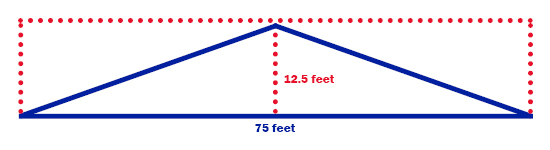# The size of the pile – Jon Urness, Vita Plus

Posted on May 26, 2016 in Forage FoundationsBy Jon Urness, Vita Plus national forage specialist
How much feed can I store in a drive-over pile given a certain area?

University extension services have some excellent tools to help you determine how much feed can be stored in a drive-over pile given a certain area.  Vita Plus also has a very comprehensive Feed Inventory Projector Program.

But sometimes, really simple geometry can get you the answer.  It’s all predicated on determining the cubic feet of space within a pile and knowing the as-fed density of the feed in that pile.

Start by looking at a cross section of a pile from the end. The pile may be roughly the shape of a low pyramid or it may have sloping sides with a roughly flat plateau.

Pyramid shape
Let’s start with the pyramid-shaped pile.  As you look at the face, you’re actually looking at a triangle, but for the moment, think of it as a rectangle with a width and a height.  Multiply the width by the height and divide by 2.  This will give you the square footage of the pile face.

For example, if you have a 75-foot-wide pad available for silage and you want a maximum height of 12.5 feet (1:3 recommended side slope), simply multiply those two numbers and divide by 2.

Pile face square footage = (75 × 12.5) / 2 = 468.75 square feet

That’s how many square feet of area is on the face and is also the cubic feet of area per running foot of the pile.  If we can achieve 45 pounds of as-fed density, multiply that by the square footage of the pile face (468.75 square feet) and we get 21,093 pounds, or about 10.5 tons per running foot.  Now multiply that times the length of the pad and you get a nominal total pile capacity.

This is especially handy for calculating daily removal rate. The silage pile will likely not be perfectly pyramid shaped, and there will be an approach ramp on the front and back, so actual capacity will be a bit less.Flat top
What about a pile with a rather flat top?  Imagine a middle, rectangular-shaped portion flanked by two right triangles. Let’s say we have a 90-foot-wide pile with a 30-foot “plateau” in the center that maxes out at 14 feet in height. That gives us a center rectangle that is 30 feet by 14 feet (420 square feet) and two triangles 30 feet wide and 14 feet high on each side. For the triangles, because they are both the same on each side, just multiply 14 feet by 30 feet and you get the area of the combined triangles.  In this case, it is the same as the center surface area (420 sqare feet).  Add the two and we have a total face that is 840 square feet.  Once again, using 45 pounds as-fed density, multiply 45 by 840 feet to get 37,800 pounds of feed per running foot (almost 19 tons).Be cautious!
A 1:3 side slope is recommended with 1:2 being the absolute maximum for safely negotiating a drive-over pile. Maximum height will determine your slope ratio. Slope is calculated by taking the maximum height and dividing by the distance from the edge. In our plateau example above, the slope runs from the end of the pile to the beginning of the plateau and is a distance of only 30 feet. The height where the plateau begins is 14 feet, so our slope is really close to the maximum of 1:2 (30 divided by 14 equals 2.14).

This same exercise can be used very easily for bunkers. Determine an average height and multiply by width to get the face area.  Multiply that by expected or measured density and you will have capacity per running foot. Estimating 45 to 50 pounds per cubic foot is a fair starting point for as-fed density.

 Category: Forage Foundations Forage storage and management